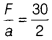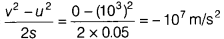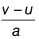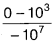Find the mass of the body

1. A force of 30 N produces an acceleration of 2 m/s2 in a body of mass m. Find the mass of the body. Also, find the acceleration, if force is doubled in magnitude along the same direction.
2. A bullet of mass 10 g strikes a sand bag with the speed of 103 m/s after covering the distance of 5 cm.
Based on above information, calculate the following:
• The resistive force exerted by sand on the bullet.
• The time taken by bullet to come to rest.
1. Given, F = 30 N, a = 2 m/s2
So, mass of body, m == 15 kg [∵ F = ma]
If f is double, a is double as F ∝ a [since, m is constant]
So, a = 4m/s2
2. Given, m = 10 g = 0.01 kg,
u = 103m/s, v = 0
s = 5 cm = 0.05 m
• Acceleration of bullet, a =[From third equation of motion]
Force applied by the bullet, F = 0.01 x -107 = - 105N [Negative sign shows direction of force against the direction of motion]
The resistive force exerted by the sand on the bullet = 105
• Time taken by bullet to come to rest, t === 10–4 [From first equation of motion]